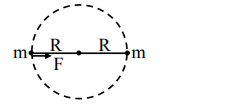# Two identical particles of mass 1 kg each go round a circle of radius R,`
Question:

Two identical particles of mass 1 kg each go round a circle of radius R, under the action of their mutual gravitational attraction. The angular speed of each particle is :

1. $\sqrt{\frac{G}{2 R^{3}}}$

2. $\frac{1}{2} \sqrt{\frac{\mathrm{G}}{\mathrm{R}^{3}}}$

3. $\frac{1}{2 R} \sqrt{\frac{1}{G}}$

4. $\sqrt{\frac{2 G}{R^{3}}}$

Correct Option: , 2

Solution:$F=\frac{\mathrm{Gm}^{2}}{(2 \mathrm{R})^{2}}=m R \omega^{2}$

$\omega=\frac{1}{2} \sqrt{\frac{G}{R^{3}}}$